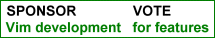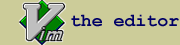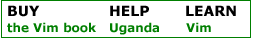increment.vim : yet another script for incrementing numbers.

 created by Srinath Avadhanula script type utility description This script provides a way to quickly create incremented lists (either increasing or decreasing) using the visual block mode. Synopsis: ========= 1. Suppose you have a column of the following form:     array(1) = 3;     array(1) = 3;     array(1) = 3;     array(1) = 3;     array(1) = 3; 2. Choose the column of '1's using Ctrl-V and then run the command Inc (you    should see "'<,'>Inc" on the command line at this point)(if you do not    have any other user commands starting with 'I', just I will suffice).    This will tranform the text as:     array(1) = 3;       array(1) = 3;     array(1) = 3;       array(2) = 3;     array(1) = 3;  --> array(3) = 3;     array(1) = 3;       array(4) = 3;     array(1) = 3;       array(5) = 3; 3. You can then choose the column of '3's (again using Ctrl-V) and run the    command "Inc 3" to generate another incremented list. This will    generate:     array(1) = 3;        array(1) =  3;     array(2) = 3;        array(2) =  6;     array(3) = 3;  -->  array(3) =  9;     array(4) = 3;        array(4) = 12;     array(5) = 3;        array(5) = 15;          Note: increment.vim automatically pads the numbers in the the column     with spaces in order to get them right aligned. This is useful in most     cases, but for cases when this might be bad, use IncN which doesnt do     any alignment. Commands provided: ================== 1. Inc : generates a column of increasing numbers with RIGHT  alignment. 2. IncN: generates a column of increasing numbers with NO     alignment. 3. IncL: generates a column of increasing numbers with LEFT   alignment Tip: A mapping which goes well with this command is the following: vnoremap :Inc With this mapping, select a column of numbers and press Ctrl-A, which will get them in increasing order. I use because its similar to the command in normal mode which increments the number under the cursor. Acknowledgements: =============== There are other scripts which have been made to do almost the same thing. Charles E Campbell's vimtip #150         uses a similar visual block strategy Stanislav Sitar's         vimscript #145     uses a searc/replace strategy install details drop the file in the vim plugins directory.

 rate this script Life Changing Helpful UnfulfillingIf you have questions or remarks about this site, visit the vimonline development pages. Please use this site responsibly. Questions about Vim should go to the maillist. Help Bram help Uganda. OSDN